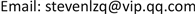1重庆大学电气工程学院，重庆

2国网新疆电力有限公司电力科学研究院，新疆 乌鲁木齐Copyright © 2019 by author(s) and Hans Publishers Inc.1. 引言

1998年美国、加拿大发生严重冰灾，加拿大东南部和魁北克南部省份的受灾情况最为严重，造成了28人死亡，470万加拿大人和50万美国人遭受停电影响  ；2008年，我国南方大范围遭遇了严重的持续低温雨雪冰冻灾害天气，大范围雨雪冰冻天气影响了我国19个省区，造成大范围倒塔、断线和闪络事故，贵州、湖南、江西和浙江省电网受损最为严重 。2018年1~2月，中国华南和华东地区普遍出现大面积的积雪冰冻天气，输电线路再次面临考验 。

2. 基于微气象监测的输电线路冰风荷载预测2.1. 输电线路冰厚预测

The icing forecast result

299.48710.98810.63214
3010.22211.00810.63214
3110.9349.76410.63214
3214.3568.98110.63214
3311.5888.78210.63214
3414.1368.46810.63214

2.2. 输电线路冰风组合荷载

m ice = 0.5 π ρ ice Δ r ⋅ r ice (1)

r ice = 0.5 Δ r + 0.25 π d (2)

q m = 9.8 a c ( d + 2 Δ r ) v 2 16 (3)

Wind speed unevenness coefficient tabl

H − H 0 = E A l 2 24 ( q 2 H 2 − q 0 2 H 0 2 ) (4)

3. 风沙磨损导致的强度损失

W sand = 0.4585 t 2 − 11.6 t + 114.4 (5)

W = 0.2712 t 2 − 7.257 t + 107.9 (6)

4. 输电线路冰风荷载失效概率评估4.1. 失效概率模型

Z ( t ) = g ( R ( t 1 ) , S ( t 2 ) ) = R ( t 1 ) − S ( t 2 ) (7)

P f ( t 1 , t 2 ) = P ( Z ( t 1 , t 2 ) < 0 ) = P ( R ( t 1 ) − S ( t 2 ) < 0 ) (8)

f R ( t 1 ) , S ( t 2 ) ( r , s ) = f R ( t 1 ) ( r ) ⋅ f S ( t 2 ) ( s ) (9)

p f ( t 1 , t 2 ) = p ( R ( t 1 ) < S ( t 2 ) ) = ∬ f R ( t 1 ) ( r ) ⋅ f S ( t 2 ) ( s ) d r d s = ∫ 0 + ∞ f S ( t 2 ) ( s ) F R ( t 2 ) ( s ) d s (10)

f Z ( t 1 , t 2 ) = 1 2 π σ Z exp ( − ( z − μ Z ( t 1 , t 2 ) ) 2 2 σ Z 2 ) (11)

(12)

α ( t 1 , t 2 ) = z ( t 1 , t 2 ) − μ Z ( t 1 , t 2 ) σ Z (13)

p f ( t 1 , t 2 ) = p ( Z ( t 1 , t 2 ) < 0 ) = ∫ − ∞ − μ z ( t 1 , t 2 ) σ Z 1 2 π exp ( − α 2 ( t 1 , t 2 ) 2 ) d α (14)

p f ( t 1 , t 2 ) = Φ ( − μ z ( t 1 , t 2 ) σ Z ) (15)

P f , eq ( t ) = 1 − ∏ i = 1 n ( 1 − P f ( i ) ) (16)

4.2. 评估流程

5. 算例分析

Conductor parameters of LGJK-310/5

6. 结语